Assertion & Reason Test: Coordinate Geometry - 1

# Assertion & Reason Test: Coordinate Geometry - 1

Test Description

## 10 Questions MCQ Test Online MCQ Tests for Class 10 | Assertion & Reason Test: Coordinate Geometry - 1

Assertion & Reason Test: Coordinate Geometry - 1 for Class 10 2022 is part of Online MCQ Tests for Class 10 preparation. The Assertion & Reason Test: Coordinate Geometry - 1 questions and answers have been prepared according to the Class 10 exam syllabus.The Assertion & Reason Test: Coordinate Geometry - 1 MCQs are made for Class 10 2022 Exam. Find important definitions, questions, notes, meanings, examples, exercises, MCQs and online tests for Assertion & Reason Test: Coordinate Geometry - 1 below.
Solutions of Assertion & Reason Test: Coordinate Geometry - 1 questions in English are available as part of our Online MCQ Tests for Class 10 for Class 10 & Assertion & Reason Test: Coordinate Geometry - 1 solutions in Hindi for Online MCQ Tests for Class 10 course. Download more important topics, notes, lectures and mock test series for Class 10 Exam by signing up for free. Attempt Assertion & Reason Test: Coordinate Geometry - 1 | 10 questions in 20 minutes | Mock test for Class 10 preparation | Free important questions MCQ to study Online MCQ Tests for Class 10 for Class 10 Exam | Download free PDF with solutions
 1 Crore+ students have signed up on EduRev. Have you?
Assertion & Reason Test: Coordinate Geometry - 1 - Question 1

### Directions: In the following questions, A statement of Assertion (A) is followed by a statement of Reason (R). Mark the correct choice as.Assertion (A): The ordinate of a point A on y-axis is 5 and B has coordinates (–3, 1). Then the length of AB is 5 units.Reason (R): The point A(2, 7) lies on the perpendicular bisector of line segment joining the points P(6, 5) and Q(0, –4).

Detailed Solution for Assertion & Reason Test: Coordinate Geometry - 1 - Question 1 In case of assertion

Here, A → (0 , 5) and B → (– 3 , 1)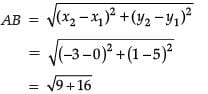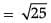= 5 units

∴ Assertion is correct.

In case of reason: If A (2, 7) lies on perpendicular bisector of P (6, 5) and Q (0, –4), then

AP = AQ

∴ By using Distance Formula,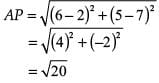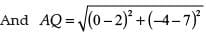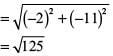As, AP ≠ AQ

Therefore, A does not lie on the perpendicular bisector of PQ.

∴ Reason is incorrect.

Hence, assertion is correct but reason is incorrect.

Assertion & Reason Test: Coordinate Geometry - 1 - Question 2

### Directions: In the following questions, A statement of Assertion (A) is followed by a statement of Reason (R). Mark the correct choice as.Assertion : The area of the triangle with vertices (-5 , -1), (3, -5), (5, 2), is 32 square units.Reason : The point (x, y) divides the line segment joining the points (x1, y1) and (x2, y2) in the ratio k : 1 externally then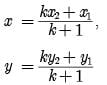Detailed Solution for Assertion & Reason Test: Coordinate Geometry - 1 - Question 2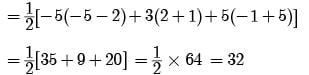and section formula (externally), we have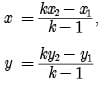Assertion & Reason Test: Coordinate Geometry - 1 - Question 3

### Directions: In the following questions, A statement of Assertion (A) is followed by a statement of Reason (R). Mark the correct choice as.Assertion : The co-ordinates of the point which divides the join of A(-5, 11) and B(4,-7) in the ratio 7 : 2 is (2, -3) Reason : The coordinates of the point P(x, y) which divides the line segment joining the points A(x1, y1) and B(x2, y2) in the ratio m1 : m2 is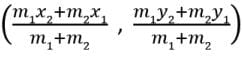Detailed Solution for Assertion & Reason Test: Coordinate Geometry - 1 - Question 3 We know that the coordinates of the point P(x, y) which divides the line segment joining the points A(x1, y1) and B(x2, y2) in the ratio m1 : m2 is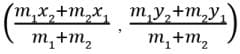So, Reason is correct.

Here, x1 = -5, y1 = 11, x2 = 4, y2 = -7, m1 = 7, m2 = 2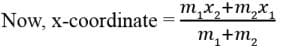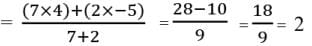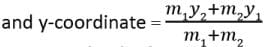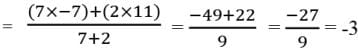So, Assertion is also correct

Assertion & Reason Test: Coordinate Geometry - 1 - Question 4

Directions: In the following questions, A statement of Assertion (A) is followed by a statement of Reason (R). Mark the correct choice as.

Assertion (A): △ABC with vertices A(–2, 0), B(2, 0) and C(0, 2) is similar to △DEF with vertices D(–4, 0), E(4, 0) and F(0, 4).

Reason (R): A circle has its centre at the origin and a point P(5, 0) lies on it. The point Q(6, 8) lies outside the circle.

Detailed Solution for Assertion & Reason Test: Coordinate Geometry - 1 - Question 4 In case of assertion: By using Distance Formula,

∴ Distance between A (-2, 0) and B (2, 0),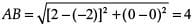[∵ distance between the points (x1, y1) and (x2, y2)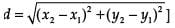Similarly, distance between B (2, 0) and C (0, 2),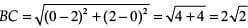In △ABC, distance between C (0, 2) and A (-2, 0),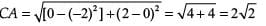Distance between F (0, 4) and D (–4, 0),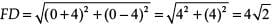Distance between F (0, 4) and E (4, 0),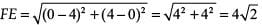and distance between E (4, 0) and D (–4, 0),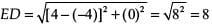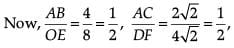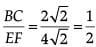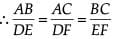Here, we see that sides of DABC and DFDE are proportional.

Therefore, by SSS similarity rule, both the triangles are similar.

∴ Assertion is correct.

In case of reason: Point Q (6, 8) will lie outside the circle if its distance from the centre of circle is greater than the radius of the circle.

Distance between centre O (0, 0) and P (5, 0),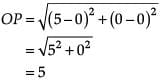As, point P lies at the circle, therefore, OP = Radius of circle.

Distance between centre O (0, 0) and Q (6, 8),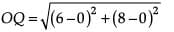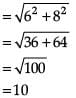As OQ > OP, therefore, point Q (6, 8) lies outside of the circle.

∴ Reason is correct.

Hence, both assertion and reason are correct but reason is not the correct explanation for assertion.

Assertion & Reason Test: Coordinate Geometry - 1 - Question 5

Directions: In the following questions, A statement of Assertion (A) is followed by a statement of Reason (R). Mark the correct choice as.

Assertion : The points (k, 2 -2k), (- k+ 1,2k) and (- 4 - k, 6 - 2k) are collinear if k = 1/2.

Reason : Three points A,B and C are collinear in same straight line, if AB + BC = AC.

Detailed Solution for Assertion & Reason Test: Coordinate Geometry - 1 - Question 5 Consider the given points.

(−k + 1, 2k), (k, 2 − 2k),(−4 −k, 6 − 2k)

Since, these points are collinear. Therefore the area of triangle formed by the triangle formed by the points will be zero.

Therefore,

(−k + 1)(2 − 2k − 6 + 2k) + k(6 − 2k − 2k) + (−4 − k)(2k − 2 + 2k) = 0

(−k + 1)(−4) + k(6 − 4k) + (−4 − k)(4k − 2) = 0

4k − 4 + 6k − 4k2 − 16k + 8 − 4k2 + 2k = 0

−2k2 − k + 1 = 0

2k2 + k − 1 = 0

2k2 + 2k − k − 1 = 0

2k(k + 1) − 1(k + 1) = 0

(k + 1)(2k − 1) = 0

k = −1 or k = 1/2.​

Assertion & Reason Test: Coordinate Geometry - 1 - Question 6

Directions: In the following questions, A statement of Assertion (A) is followed by a statement of Reason (R). Mark the correct choice as.

Assertion : If the points A(4, 3) and B(x, 5) lies on a circle with the centre O(2,3) then the value of x is 2.

Reason : The mid-point of the line segment joining the points P(x1, y1) and Q(x2, y2) is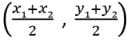Detailed Solution for Assertion & Reason Test: Coordinate Geometry - 1 - Question 6 We know that the mid-point of the line segment joining the points P(x1, y1) and Q(x2, y2) is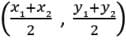So, Reason is correct.

Given, the points A (4,3) and B (x, 5) lie on a circle with center O(2,3).

Then OA = OB ⇒(OA)2 = (OB)2

⇒ (4 – 2)2 + (3 – 3)2 = (x – 2)2 + (5 – 3)2

⇒ (2)2 +(0)2 = (x – 2)2 + (2)2 ⇒ 4 = (x – 2)2 + 4 ⇒(x – 2)2 = 0

⇒ x – 2 = 0 ⇒ x = 2

So, Assertion is correct

Assertion & Reason Test: Coordinate Geometry - 1 - Question 7

Directions: In the following questions, A statement of Assertion (A) is followed by a statement of Reason (R). Mark the correct choice as.

Assertion (A): If the distance between the point (4, p) and (1, 0) is 5, then the value of p is 4.

Reason (R): The point which divides the line segment joining the points (7, – 6) and (3, 4) in ratio 1 : 2 internally lies in the fourth quadrant.

Detailed Solution for Assertion & Reason Test: Coordinate Geometry - 1 - Question 7 In case of assertion: Distance between two points (x1, y1) and (x2, y2) is given as,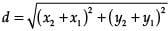where, (x1, y1) = (4, p)

(x2, y2) = (1, 0)

And, d = 5

Put the values, we have

52 = (1 − 4)2 + (0 – p)2

25 = (–3)2 + (–p)2

25 – 9 = p2

16 = p2

+4, –4 = p

∴ Assertion is incorrect.

In case of reason:

Let (x, y) be the point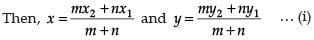Here, x1 = 7, y1 = –6, x2 = 3, y2 = 4, m = 1 and n = 2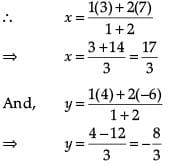So, the required point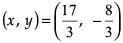lies in IVth quadrant.

∴ Reason is correct.

Hence, assertion is incorrect but reason is correct.

Assertion & Reason Test: Coordinate Geometry - 1 - Question 8

Directions: In the following questions, A statement of Assertion (A) is followed by a statement of Reason (R). Mark the correct choice as.

Assertion : Centroid of a triangle formed by the points (a, b) (b, c), and (c, a) is at origin, Then a + b + c = 0 .

Reason : Centroid of a △ABC with vertices A (x1, y1), B(x2, y2) and C (x3, y3) is given by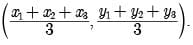Detailed Solution for Assertion & Reason Test: Coordinate Geometry - 1 - Question 8 Centroid of a triangle with vertices (a, b) (b, c), and (c, a) is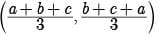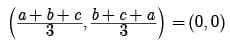a + b + c = 0

Assertion & Reason Test: Coordinate Geometry - 1 - Question 9

Directions: In the following questions, A statement of Assertion (A) is followed by a statement of Reason (R). Mark the correct choice as.

Assertion : The possible values of x for which the distance between the points A(x, -1) and B(5, 3) is 5 units are 2 and 8.

Reason : Distance between two given points A(x1, y1) and B(x2, y2) is given by,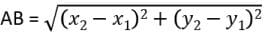Detailed Solution for Assertion & Reason Test: Coordinate Geometry - 1 - Question 9 We know that the Distance between two given points A(x1, y1) and B(x2, y2) is given by,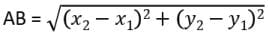So, Reason is correct.

Now, AB = 5 ⇒ AB2 = 25

⇒ (x – 5)2 + (-1 - 3)2 = 25

⇒ (x – 5)2 = 25 – 16 = 9

⇒ x – 5 = ±3

⇒ x = 5 ± 3

⇒ x = 2, 8

So, Assertion is also correct

Assertion & Reason Test: Coordinate Geometry - 1 - Question 10

Directions: In the following questions, A statement of Assertion (A) is followed by a statement of Reason (R). Mark the correct choice as.

Assertion : Mid-point of a line segment divides line in the ratio 1 : 1.

Reason : If area of triangle is zero that means points are collinear.

Detailed Solution for Assertion & Reason Test: Coordinate Geometry - 1 - Question 10 Let us assume that point B divides the line AC in the ratio k : 1

Let(x1, y1, z1) = (0, 0, 0)

(x, y ,z) = (2, −3, 3)

(x2, y2, z2) = (−2, 3, −3)

By section formula:

x = m1 + m2 m1 x 2 + m2 x 1

⇒ 2 = k + 1 k.(−2) + 1.(0)

⇒ k = 4 − 1 on neglecting the negative sign

we get, therefore ratio is 1:4.

## Online MCQ Tests for Class 10

456 tests
 Use Code STAYHOME200 and get INR 200 additional OFF Use Coupon Code
Information about Assertion & Reason Test: Coordinate Geometry - 1 Page
In this test you can find the Exam questions for Assertion & Reason Test: Coordinate Geometry - 1 solved & explained in the simplest way possible. Besides giving Questions and answers for Assertion & Reason Test: Coordinate Geometry - 1, EduRev gives you an ample number of Online tests for practice

456 tests